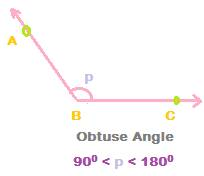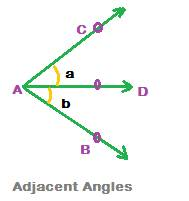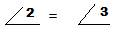## Various Types of Angles

1. Acute angle:An angle whose measure is from 00 to 900 is called an acute angle.

If x is an acute angle, then x < 900

2. Right angle:An angle whose measure is 900 is called a Right angle.

The square symbol denotes right angle, i.e. 900

If p is a right angle, then /p = 900

The two straight lines AB and AC are called perpendicular lines. It is denoted as below:

AB │ AC

Angle between two lines which are perpendicular to each other is 900

3. Obtuse angleAn angle whose measure is from 900 to 1800 is called an obtuse angle.

If p is an obtuse angle, then p can be written as: 900 < p < 1800

4. Reflex angleAn angle whose measure is from 1800 to 3600 is called a reflex angle.

Let ‘a’ denote a reflex angle. Then, ‘a’ can be written as

1800 < a < 3600

In the figure above for reflex angle, initial ray AB is rotated anticlockwise to reach the final ray position AC.

Reflex angle is formed when the initial ray AB is rotated beyond a straight line but within a full round.

5. Straight angleAn angle whose measure is 1800 is called a straight angle.

Rotate initial ray AB anti clockwise so that its position on AC, the final ray, points exactly in opposite direction of initial ray AB.Two angles that have a common vertex and arm are called adjacent angles.

In the above figure, angles a and b are adjacent angles.

The two angles a and b have a common vertex A and a common side AD.

7. Linear Pair of anglesTwo adjacent angles whose sum is 1800 are called linear pair of angles.

In the above figure, angles x and y are a pair of adjacent angles.

The sum of the measures of the two adjacent angles x and y is 1800.Therefore x and y form a linear pair of angles.

Note:

All linear pair of angles are adjacent angles, but

All adjacent angles are not linear pair of angles.

8. Vertically opposite anglesTwo straight lines that intersect at a point form angles which are called vertically opposite angles.

Angles in the above figure are denoted by numerals 1, 2, 3 and 4.

The two straight lines AD and BC intersect each other at point P.

P is called the point of intersection of the two straight lines AD and BC.

In the above figure, there are two pairs of vertically opposite angles. One pair is angles 1 and 4. The other pair is angles 2 and 3.

Now,and alsoAlso note that:andand9. Complementary anglesTwo angles whose sum is 900 are called complementary angles.

In the figure aboveNote:

If p is one angle, then its complement is 900 – p

10. Supplementary anglesTwo angles whose sum is 1800 are called supplementary angles.

In the above figure, angles 1 and 2 are supplementary angles.Also, they form a linear pair of angles.

Note:

If x is one angle, then its supplement is 1800 – x

11. What is the sum of all of the angles at a point?In the above figure, the three straight lines AC, AB and AD intersect each other at point A.

Around vertex A, three angles are formed. They are named X, Y and Z.

Now,